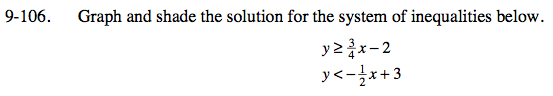### Home > CCA > Chapter 9 > Lesson 9.4.2 > Problem9-106

9-106.
1. Graph and shade the solution for the system of inequalities below. 9-106 HW eTool (Desmos). ​ Homework Help ✎

2. yx − 2
y < −x + 3Graph the boundary line for each inequality.

For both lines, test a point by inputing the coordinates into the equation. If the point makes the equation true, shade the side of the line containing that point. If the point makes the equation false, shade the opposite side. The answer to the system of inequalities is the region of overlap.

Use the eTool below to complete graph for the problem.
Click the link at right for the full eTool version: CCA 9-106 HW eTool